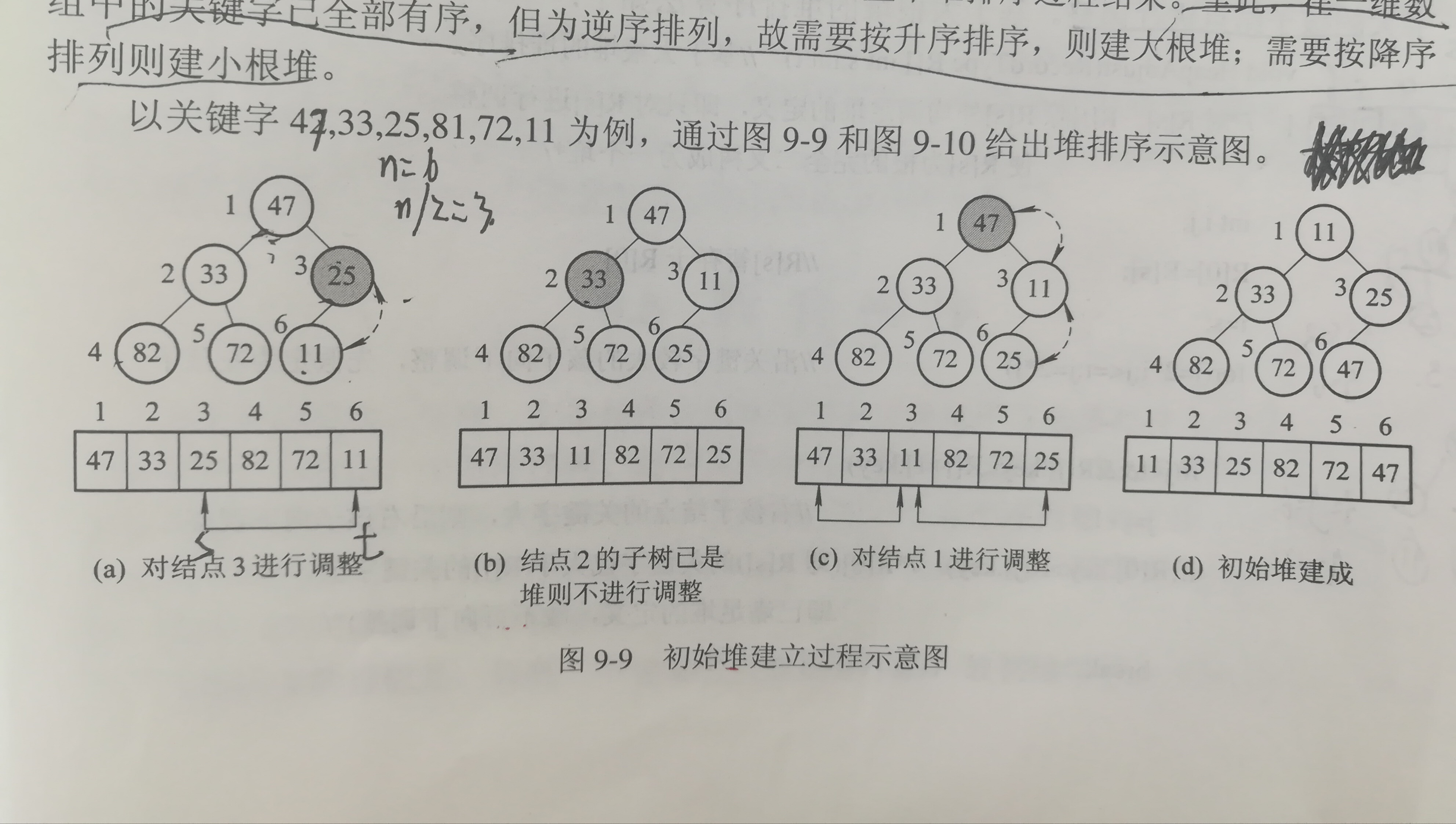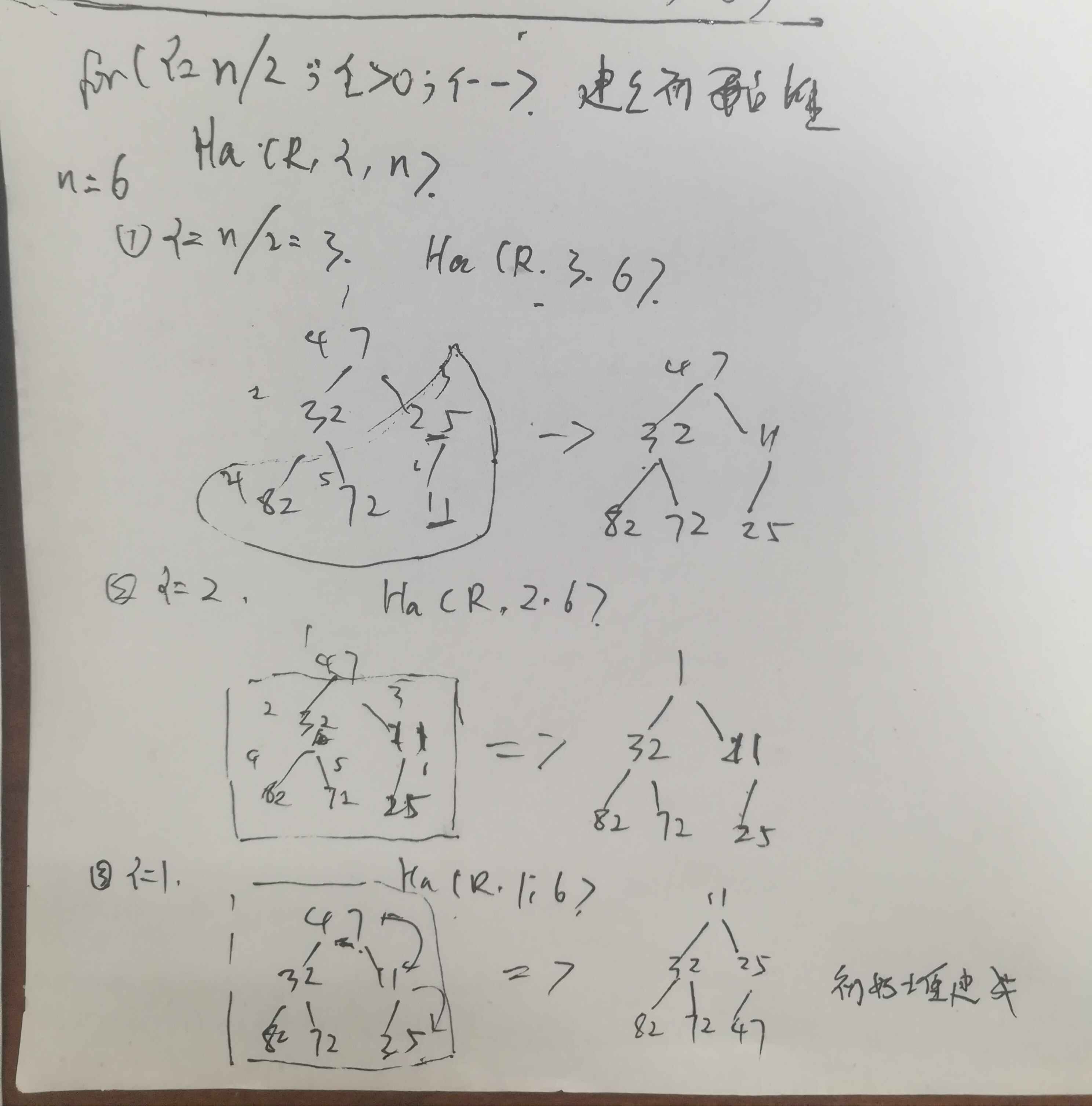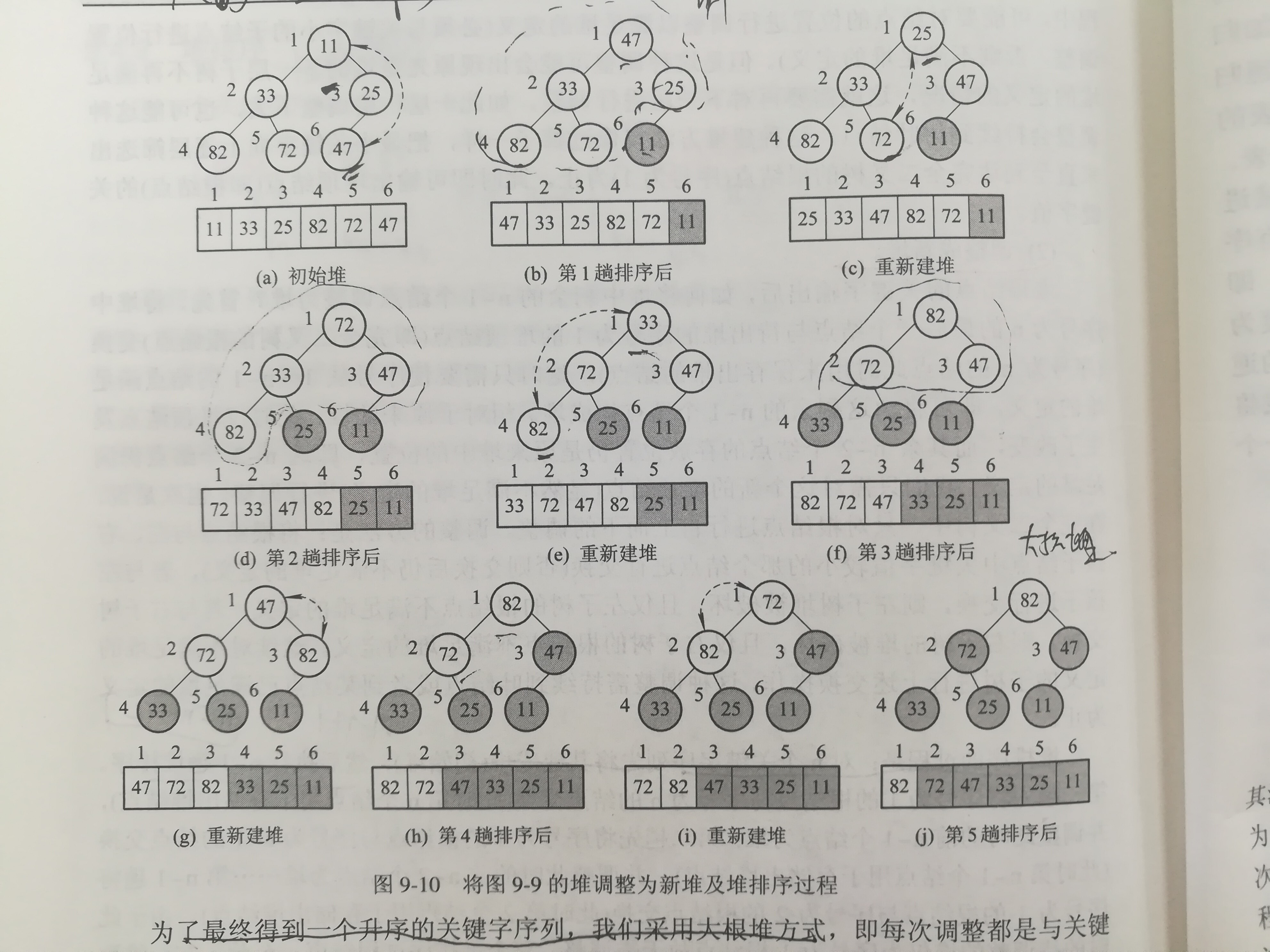# 冒泡排序，选择排序，快速排序，堆排序详解

A,冒泡排序
/**
* 冒泡排序：每次将相邻两个数比较，如果不满足排序条件则交换位置
* 比较次数：k>=n-1&&k<=(1/2)n(n-1)
* 时间复杂度：O(n)<T(n)<O(n^2)
*/
public class BubbleSort {
public static void bubbleSort(int[] a) {
for (int i = 0; i < a.length - 1; i++)//控制排序趟数:最多n-1趟.
{
int swap = 0;//设置交换标志
for (int j = 0; j < a.length - i - 1; j++)//控制比较次数。升序：每趟每次将相邻两个数比较,每趟从第n-i个到第n个元素已为升序排列
{
if (a[j] > a[j + 1])//交换
{
int temp = a[j];
a[j] = a[j + 1];
a[j + 1] = temp;
swap = 1;//有交换发生
}
}//一趟完毕后，从第一个元素开始，每相邻两个元素，前一个元素小于后一个元素，即a[2k]>a[2k+1](k=1,2,3...n/2-1)
if (swap == 0)//本趟已有序,结束排序
{
break;
}
}//外层for结束
}//方法bubbleSort结束
}
B,选择排序
/**
* 选择排序
* 基本思想：
*      每次从待排序记录中选择出关键字最小的记录，并顺序放在已排好序记录序列的最后，
*      直到全部记录序列排序完成为止。
* 适用场景：
*      由于选择排序每趟总是从无序记录中跳出关键字最小的记录，因此适合从大量记录中选择一部分记录的场景。
*  如从10000个记录中选择出关键字最小或最大的前10个记录，就适宜采用选择排序。
*  实现方法：
*      第一趟从n个无序记录中选出关键字最小的记录与第一个记录交换(此时第一个记录已有序）；
* 第二趟再从第二个记录开始的n-1个记录中选择出关键字最小的记录与第二个记录交换（此时前二个记录已有序）....
* 如此下去，第i趟则从第i个记录开始的n-i+1个记录中选出关键字最小的记录与第i个记录交换 （此时前i个记录已有序），
* 这样n-1趟后前n-1个记录已有序，无序记录只剩一个即第n个记录，因此关键字小的前n-1个记录已进入有序序列，
* 这第n个记录比为关键字最大的记录，所以无需交换即n个记录已全部有序。
* 时间复杂度：
* 总比较次数：i:[1:n-1]*(n-i)=(1/2)n(n-1)]
* 因此时间复杂度为：O（n^2)
* 最好情况下是n个记录一开始即为有序，移动次数为0，
* 最坏情况下是初始的n个记录为逆序排列，即每一趟都要执行交换操作，总的移动次数为3（n-1)
* 所以直接选择排序是一种不稳定的排序方法
*
*/
public class SelectSort {
/**
* 直接选择排序算法：
* @param r 待排序的记录数组
* @param n 要排序的记录个数
*/
public static void selectSort(int r[],int n){
//对R~R[n]这n个记录进行选择排序
int i,j,k;
for(i=1;i<n;i++){
k=i;
for(j=i+1;j<=n;j++){
if(r[j]<r[k]){
k=j;//保存关键字最小的记录的存放位置
}
}
if(i!=j){//将找到关键字最小的记录与第i个记录交换
r=r[k];
r[k]=r[i];
r[i]=r;
}
}
}
}

C,快速排序
/**
* 快速排序：核心算法：划分。
* 时间复杂度：
* O（nlogn)<=T(n)<=O(n^2)
* 排序过程：
* 任取其中一个记录（通常是第一个记录）为基准，
* 经过一趟交换之后，所有关键字比它小的记录都交换到它的左边，所有关键字比它大的都交换到它的右边，
* 此时，基准记录在序列中的最终位置就已经确定。
* 然后再分别对划分到基准记录左右两部分区间的记录序列重复上述过程，直到每一部分最终划分为一个记录时为止。
*/
public class QuickSort {

//划分算法：设置两个搜索指针i,j。起始时，i指向给定区间的第一个一个元素，j指向最后一个元素。对每个区间进行划分。
public static int Partition(int[] a, int i, int j) {
int key = a[i];
while (i < j)//控制排序趟数，当i>=j时，一趟排序完成
{//每趟排序的扫描，交换过程
while (i < j && a[j] >= key)//当j找到的是比a元素时，继续向前扫描，直到找到小于a的元素后停止移动
{
j--;//j左移一位
}
if (i < j)//再次判断i,j的位置，
{
int temp = a[i];//交换a[i]和a[j]
a[i] = a[j];
a[j] = temp;
i++;//i右移一位,因为i刚才的位置上的元素已经和j交换，存的是小于a的元素，i向右移一位，到下一个元素，继续和a[0】比较
}
while (i < j && a[i] <= key)//i自左向右移动，直到找到大于a的元素时，停止移动
{
i++;//i右移一位
}
if (i < j)//再次判断i,j的位置，
{
int temp = a[j];//交换a[i]和a[j]
a[j] = a[i];
a[i] = temp;
j--;//j左移一位,因为j刚才的位置上的元素已经和a[i]交换，存的是大于a的元素，j向左移一位，到下一个元素，继续和a[0】比较
}
}//while循环结束，一趟排序完成
return i;//返回基准记录a最终存放的位置。便于确定下次划分位置。
}//方法Partition结束

public static void QuickSort(int[] a, int s, int r) {
int i;
if (s < r) {
i = Partition(a, s, r);//本趟结束后，基准记录的最终位置
QuickSort(a, s, i - 1);//对基准记录的左区间再次进行划分
QuickSort(a, i + 1, r);//对基准记录的右区间再次进行划分
}
}
}
D,堆排序
package wjh.mysort;

/**
* 堆排序：
* 堆排序是一种树形选择排序，更确切的说是一种树形选择排序。
* 堆分为大根堆和小根堆
* 大根堆：父节点大于子节点的值
* 小根堆：父节点小于子节点的值
*
* 堆排序思想：
* （以小根堆为例）
* 对n个待排序的记录，首先根据各记录的关键字按堆的定义排成一个序列（即建立初始堆），从而由堆顶得到关键字最小
* 的记录，然后将剩余的n-1个记录再调整成一个新堆，即又由堆顶得到关键字最小的记录，然后将剩余的n-1个记录再调整成一个新堆，
* 即又由堆顶得到这n-1个记录中最小关键字的记录，如此反复进行出堆和将剩余记录调整为堆的过程，当堆仅剩一个记录出堆时，则n个记录已按
* 出堆次序排成有序序列。
*
* 堆排序过程：
* （1）建立初始堆
* （2）调整成新堆
* 具体过程：
*      对n个关键字序列先将其建成堆（初始堆），然后执行n-1趟堆排序，第一趟先将序号为1的根节点与序号为n的节点进行交换
* （此时第n个节点用于存储出堆节点），并调整此时的前n-1个节点为堆；第二趟将序号为1的根节点与序号为n-1的节点进行交换
* （此时第n-1个节点用于存储出堆节点），并调整此时的前n-2个节点为堆......第n-1趟将序号为1的根节点与序号为2(因为（n-(n-1)+1)=2)
* 的节点进行交换（此时第2个节点用于存储出堆节点）。由于此时待调整的对仅为序号为1的根节点故无需调整，整个堆排序过程结束。
* 至此。在一维数组中的关键字已全部有序，但为逆序排列，故需要按升序排列，则建大根堆，需要按降序排列则建小根堆。
*
* 升序需要建立大根堆，降序需要建立小根堆。
*我的理解：大根堆排序时出堆的时候是每次选最小的记录出堆，这样出堆的顺序就为升序，所以如果需要按升序排列就需要建立大根堆。
* 小根堆相反。
*
* 适用场景：
*      由于初始建堆所需比较的次数较多，因此堆排序不适合记录较少的情况。
*      对大量记录的排序来说堆排序是非常有效的。
*      并且,堆排序只需要一个记录的辅助空间（r),即其空间复杂度为O(1)。
*
* 堆排序是一种不稳定的排序算法。
* 最坏情况下时间复杂度为O（nlogn),所以在最坏情况下堆排序的时间复杂度要低于快速排序。
*/
public class HelpSort {
/**
* 为了最终得到一个升序的关键字序列，采用大根堆的方式，即每次都是与关键字大的孩子节点进行调整。
* 这是基于大根堆的排序算法：
*调整完毕之后为一个大根堆
* @param r 要排序的记录的数组
* @param s 开始下标
* @param t 结束下标
*/
public static void heapAdjust(int r[], int s, int t) {
/*对r[s]~r[t]之外的记录均满足堆的定义，即只对r[s]进行调整
* 使r[s]为根的完全二叉树成为一个堆*/
int i, j;
r = r[s]; //用r暂存r[s],r用来存放记录
i = s;
for (j = 2 * i; j <= t; j = 2 * j) {//沿关键字较大的记录向下调整，先假定为左孩子，2i为偶数即代表左孩子。
if (j < t && r[j] < r[j + 1]) {//若左孩子的值小于右孩子的值
j = j + 1;                  //则沿右孩子向下调整
}
if (r > r[j]) {//r即r[s],若r[s]已经大于r[j]的值，则已满足堆的定义，不用再向下调整
break;
}
r[i]=r[j];//将关键字大的孩子节点r[j]调整至双亲节点r[i]
i=j;//定位于孩子节点继续向下调整
}
r[i]=r;//r[i]相当于r[j].将r即r[s]调整到r[j]
}

/**
* 堆排序算法
* @param r 待排序的记录数组
* @param n 待排序的记录数
*/
public static void heapSort(int r[],int n){
int i;
for(i=n/2;i>0;i--){//建立初始堆
}
for(i=n;i>1;i--){  //对初始堆进行n-1趟堆排序
r=r;//堆顶的r与堆底的r[i]进行交换
r=r[i];
r[i]=r;
}
}
}